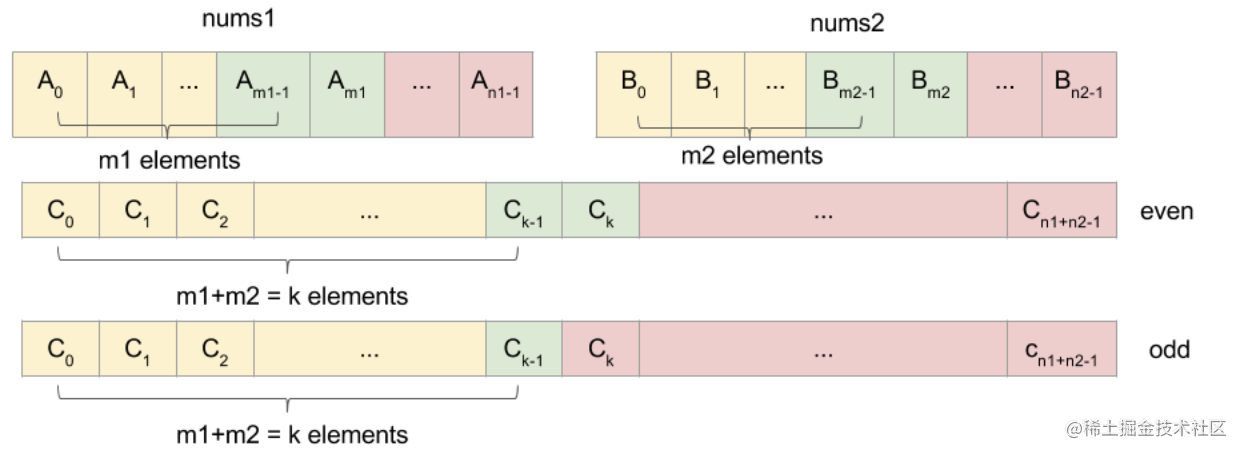# LeetCode 4 Median of Two Sorted Arrays(Tag:Array Difficulty:Hard)

## 题解

1. 暴力解法，将两个有序数组组合在一起，根据组合后数组的长度来计算其中位数，时间复杂度为 O(n)
``````/**
* @param {number[]} nums1
* @param {number[]} nums2
* @return {number}
*/
var findMedianSortedArrays = function (nums1, nums2) {
let nums = []
let i = 0
let j = 0
let k = 0
while (i < nums1.length && j < nums2.length) {
if (nums1[i] < nums2[j]) {
nums[k++] = nums1[i++]
} else {
nums[k++] = nums2[j++]
}
}
// nums1数组还有剩余元素
if (i < nums1.length) {
nums.push(...nums1.slice(i))
}
// nusm2数组还有剩余元素
if (j < nums2.length) {
nums.push(...nums2.slice(j))
}
let res
let len = nums.length
if (len % 2) {
res = nums[Math.floor(len / 2)]
} else {
res = (nums[len / 2] + nums[(len / 2) - 1]) * 0.5
}
return res

2、利用有序数组的特性来进行中位数查找，在LeetCode 数组类型题目做前必看一文中，有提到，如果题目中说到有序数组，那么应该首先想到二分搜索，这道题确实可以用二分搜索来解决，关键在于如何将其转换为二分搜索的问题。(备注：图来自 花花酱leetcode)

``````/**
* @param {number[]} nums1
* @param {number[]} nums2
* @return {number}
*/
var findMedianSortedArrays = function (nums1, nums2) {
const n1 = nums1.length;
const n2 = nums2.length;
if (n1 > n2) {
return findMedianSortedArrays(nums2, nums1);
}

const k = Math.floor((n1 + n2 + 1) / 2); // 合并后数组一半的数
let l = 0;
let r = n1;
while (l < r) {
const m1 = Math.floor((r - l) / 2) + l;
const m2 = k - m1;
if (nums1[m1] >= nums2[m2 - 1]) {
r = m1;
} else {
l = m1 + 1;
}
}

const m1 = l;
const m2 = k - l;

// k-1 位置上的取值
const c1 = Math.max(
m1 <= 0 ? -Number.MAX_VALUE : nums1[m1 - 1],
m2 <= 0 ? -Number.MAX_VALUE : nums2[m2 - 1]
);

// 合并后的数组为奇数
if ((n1 + n2) % 2 === 1) {
return c1;
}

// k 位置上的取值
const c2 = Math.min(
m1 >= n1 ? Number.MAX_VALUE : nums1[m1],
m2 >= n2 ? Number.MAX_VALUE : nums2[m2]
);

// 合并后的数组为偶数
return (c1 + c2) / 2;
};